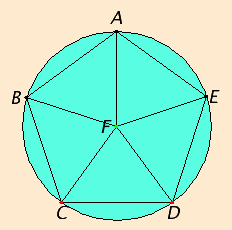# Proposition 14

To circumscribe a circle about a given equilateral and equiangular pentagon.

Let ABCDE be the given pentagon, which is equilateral and equiangular.

It is required to circumscribe a circle about the pentagon ABCDE.

I.9

Bisect the angles BCD and CDE by the straight lines CF and DF respectively. Join the straight lines FB, FA, and FE from the point F at which the straight lines meet to the points B, A, and E.As in IV.13

Then in manner similar to the preceding it can be proved that the angles CBA, BAE, and AED are also bisected by the straight lines FB, FA, and FE respectively.

I.6

Now, since the angle BCD equals the angle CDE, and the angle FCD is half of the angle BCD, and the angle CDF half of the angle CDE, therefore the angle FCD also equals the angle CDF, so that the side FC also equals the side FD.

Similarly it can be proved that each of the straight lines FB, FA, and FE also equals each of the straight lines FC and FD. Therefore the five straight lines FA, FB, FC, FD, and FE equal one another.

Therefore the circle described with center F and radius one of the straight lines FA, FB, FC, FD, or FE also passes through the remaining points, and is circumscribed.

Let it be circumscribed, and let it be ABCDE.

Therefore a circle has been circumscribed about the given equilateral and equiangular pentagon.

Q.E.F.

## Guide

The method given here to circumscribe a circle about any regular n-gon. Simply bisect two of the angles of the n-gon to find the center of the circle, then choose one of the lines from it to a vertex as the radius.

This construction is used in propositions XIII.8 and XIII.18.